Courses
Courses for Kids
Free study material
Offline Centres
More

# Adding and Subtracting Larger NumbersLast updated date: 04th Dec 2023
Total views: 111.6k
Views today: 1.11k## An Introduction to Adding and Subtracting Large Numbers

Everyone learns to count numbers at a young age, beginning with a single digit and progressing to seven digits. Adding and subtracting larger numbers is an easy maths problem that everyone can do. It involves adding or subtracting the value of one number from another. The problem is that we often write “1+1=2” without considering the actual number.

We add the same way we add other numbers. The integers are arranged in a column based on their place values. The addition procedure starts with the one's column, then moves on to the tens column, then the hundreds column, and so on. For subtraction, we do the same. This article will teach us about the place value addition and subtraction of larger numbers.

## International Place Value Chart

The International Numerical System includes tens, hundreds, thousands, ten thousand, hundred thousand, millions, etc. This can be understood from the image shown below.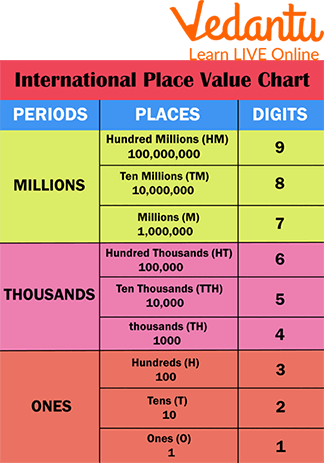International Place Value Chart

## Indian Place Value Chart

The Indian place value chart is a table that is used to find the value of each digit in a number based on its position, as per the Indian Numeral System. The place value of a number shows how much a digit is worth based on its position. In order to avoid confusion while reading very large numbers, we arrange the numbers into periods with the help of a comma as the separator.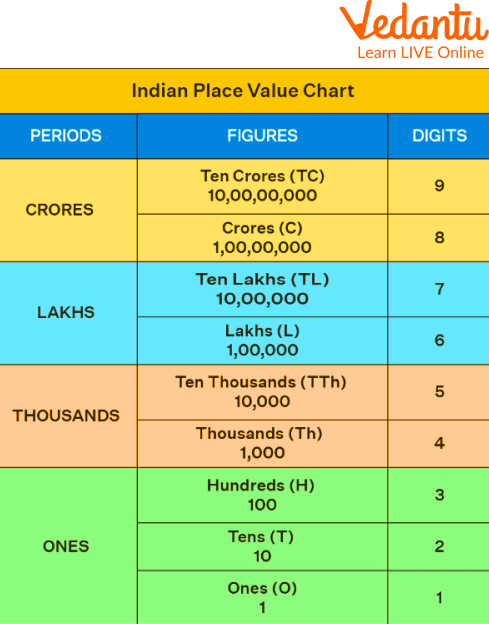Indian Place Value Chart

## Relationship Between Place Values

The place value of digits in numbers can also be represented using base-ten blocks and can help us write numbers in their expanded form.

• The hundreds place is one position to the left of the tens place and is 10 times bigger than the tens place.

• When we move one spot to the left side from the ones place, the tens place becomes 10 times greater than the ones place.

• The thousands place is 10 times bigger than the hundreds place when we go from one spot to the left side from the hundreds place.Place Value Relationship

Now observe that the value of the base 10 blocks on the far left is 3,000. The value of the blocks decreases by a factor of ten each time we walk to the right. The 3, therefore, has a value that is a tenth that of the earlier number.Place Value Relationship

## Place Value Addition and Subtraction

The addition procedure starts with the one's column, moves on to the tens column, then the hundreds column, and so on. The numbers that must be carried forward are inserted alongside the existing numbers in the adjacent column. This process must be repeated until we reach the last column and obtain our final number.

The same column order used for addition is used for large-number subtraction. Once the numbers are in the columns, we start with one and work our way to the left. Numbers are borrowed from the left side when needed.

## Adding and Subtracting with Regrouping

Regrouping happens whenever a column's sum is greater than 9. If any digit in the minuend is less than the corresponding digit in the subtrahend, regrouping is performed in subtraction. Before learning about subtraction with regrouping, let's first study addition with regrouping.

## Regrouping During Subtracting

Any time a digit in the minuend is smaller than the equivalent digit in the subtrahend, subtraction with regrouping is performed. To make the minuend digit greater than the subtrahend digit, we borrow 1 from the column before and add it to this minuend.

In addition to regrouping, when a total in any of the columns is greater than 9, we take the extra digit over to the column before it and add it together with the addends.

## Solved Examples of Adding and Subtracting Larger Numbers

Here, a few examples related to adding and subtracting larger numbers have been described.

Examples: Perform subtraction of the numbers 432 - 256.

Ans: Let's subtract these integers using the borrow method.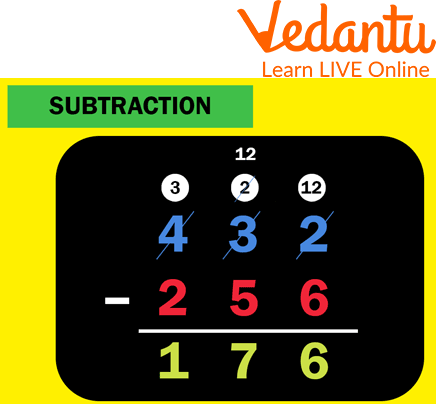Subtraction

Example: Find the sum of 355 and 466.

Ans: The sum of 355 and 466 is 821.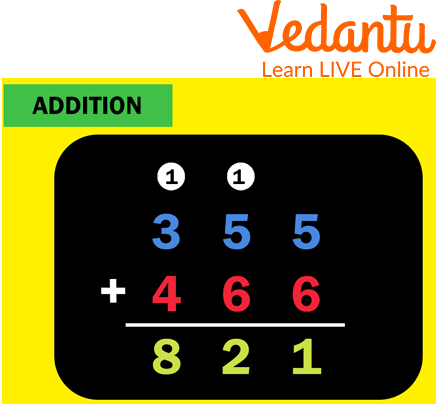## Worksheet: Adding and Subtracting Larger Numbers

Here is a worksheet related to adding and subtracting larger numbers for practice.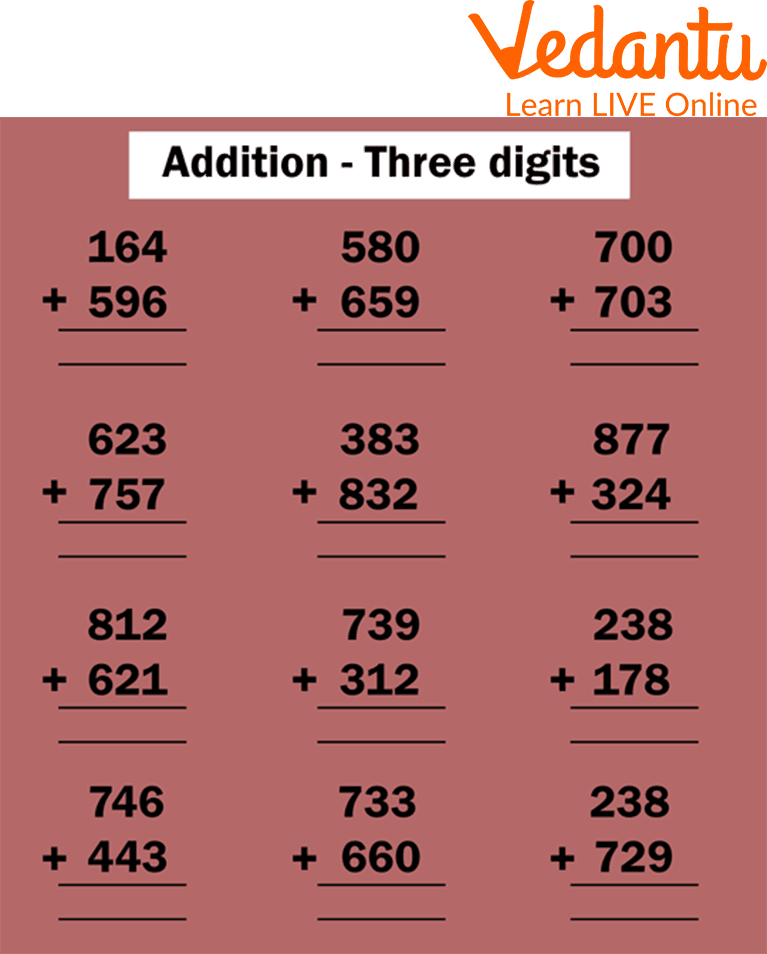Worksheet on the Addition of Three-digit Numbers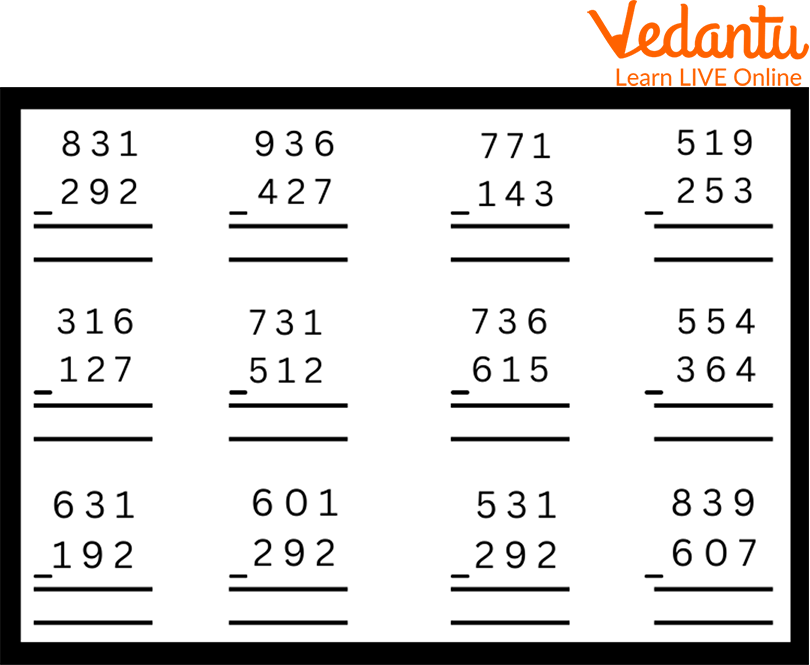Worksheet on Subtraction of Three-digit

## FAQs on Adding and Subtracting Larger Numbers

Q1. How is the subtraction of larger numbers taught?

Ans: For larger number subtraction, the following points should be taken care of:

• Place the larger number above the smaller one.

• Sort the integers into columns based on place value by stacking the digits.

• Regroup as necessary.

• Start with the column on the right and subtract while moving left.

Q2. What are a few examples of larger numbers?

Ans: Large numbers in the number system are typically bigger or greater than the other numbers. For instance, large figures like 1 lakh, 1 million, 1 billion, etc., are not ones we use frequently.

Q3. What actions must be taken to add larger numbers?

Ans: For larger number addition, the  following steps should be considered:

• The integers are stacked in place value order.

• Starting with the column on the right, add the values in the columns.

• As necessary, carry numbers into the place value adjacent to the left.

• For the last three columns, repeat this pattern.